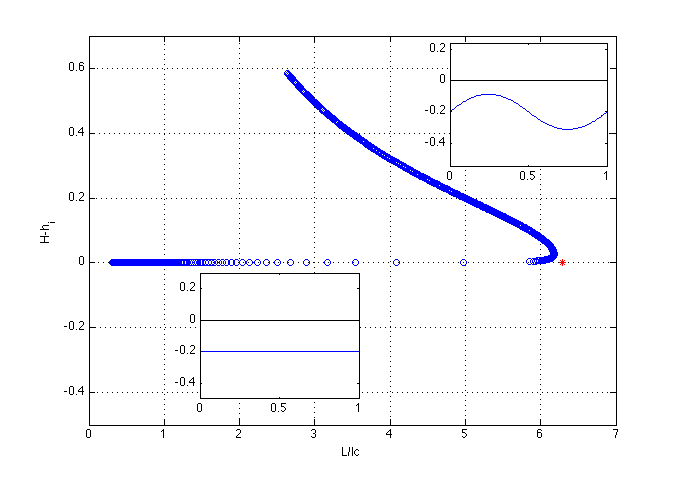# The Rayleigh-Taylor instability

This code investigates the Rayleigh-Taylor instability of a heavier thin film above a lighter one. This situation arises for example at the lower interface of a vertical tube filled with a liquid heavier than the sourrounding one. Depending on the distance between the pipe walls, the interface may be stable or unstable.

This problem is very similar to the one presented in pendant_drop_volume.m. For this reason, only the differences are highlited and the reader is referred to the outer code for more details. (The same subsections are used to facilitate the comparison).

clear all; clf;

% parameters
L=1;             % domain length
N=100;           % number of grid points
rho=1;           % density
sig=10;          % surface tension
g=-1;            % gravity
lc = sqrt(abs(sig/(rho*g))); % capillary length
hi = 0.2;        % thickness of uniform film
V=hi*L;          % volume of uniform film
delta=0.02;      % continuation length

% Differentiation and integration
[D,DD,ws,s]=dif1D('cheb',0,L,N,3);
Z=zeros(N,N); I=eye(N);


# Boundary conditions

The boundary conditions are as for the pendant drop, Dirichlet boundary conditions. However note that the vertical position of the extremities of the interface is slightly perturbed. This is necessary to find the imperfect pitchfork bifurcation.

% Boundary conditions
x1=0; xn=L;
y1=hi-0.001; yn=hi+0.001;


In the solution vector, the homotopy parameter for this problem is the surface tension \sigma. Note the negative sign for the direction of the variation of \sigma to decrease its value initially.

%initial guess
Pinit=V/L*rho*g;
initguess_x=s*L;
initguess_y=linspace(y1,yn,N)';
linit=sum(sqrt(diff(initguess_x).^2+diff(initguess_y).^2));
sol=[initguess_x;initguess_y;Pinit;linit;sig];
dir=[zeros(N,1);zeros(N,1);0;0;-1];   % initial direction

disp('Continuation loop')
ind=1;quitcon=0;
while ~quitcon
solprev=sol;


# Keller’s pseudo-arclength continuation

    sol=sol+dir*delta; % new prediction of solution

% Newton iterations
disp('Newton loop')
quit=0;count=0;
while ~quit


# Newton loop

        % the present solution and its derivatives
x=sol(1:N); y=sol(N+1:2*N); P=sol(2*N+1); l=sol(2*N+2); sig=sol(end); xp=D*x;  xpp=DD*x; yp=D*y;  ypp=DD*y; a=xp.^2+yp.^2;

% nonlinear function
f=[-rho*g*y.*a.^1.5-sig.*(xpp.*yp-ypp.*xp)+P*a.^1.5; ...
a-l^2; ...
ws*(y.*xp)-V; ...
x(1)-x1; ...
dir'*(sol-solprev)-delta];


# Computing the Jacobian

The Jacobian is computed in the same way as in the continuation over the volume. Note however the difference in the component related to the governing equation and in the one of the volume constraint because of the perturbation of the surface tension and not of the volume.

            % analytical jacobian
A=[ 3*P*diag(a.^0.5.*xp)*D-3*rho*g*diag(y.*xp.*a.^0.5)*D-sig*diag(yp)*DD+sig*diag(ypp)*D,...
3*P*diag(a.^0.5.*yp)*D-3*rho*g*diag(y.*yp.*a.^0.5)*D+sig*diag(xp)*DD-sig*diag(xpp)*D-rho*g*diag(a.^1.5),...
a.^1.5,zeros(N,1),-(xpp.*yp-ypp.*xp); ...
2*diag(xp)*D,2*diag(yp)*D,zeros(N,1),-2*l*ones(N,1),zeros(N,1); ...
y'.*I(N,:)-y'.*I(1,:)-ws.*yp',ws.*xp',0,0,0;...
I(1,:),zeros(1,N),0,0,0; ...
dir'];

% Boundary conditions
loc = [1 N N+1];
f(loc)=[x(N)-xn; y(1)-y1; y(N)-yn];
A(loc,:)=[I(N,:),zeros(1,N),0,0,0; zeros(1,N),I(1,:),0,0,0; zeros(1,N),I(N,:),0,0,0];

% convergence test
res=norm(f);
%plot(hx,hy,'b-',initguess_x,initguess_y,'r--'); drawnow;
disp([num2str(count) '  ' num2str(res)]);
if count>50|res>1e5; disp('no convergence'); endLoop =1; quitcon=1; break; end
if res<1e-5; quit=1; disp('converged'); continue; end


# The Newton step

            % Newton step
sol=sol-A\f;
count=count+1;
end


# The new direction for the continuation

    % New direction
dir=A\[zeros(N,1);zeros(N,1);0;0;1]; % new direction
dir=dir/max(abs(dir)); % normalization
max(abs(dir))
ind=ind+1;


# Bifurcation diagram and Rayleigh-Taylor instability

When reducing the surface tension the capillary length, i.e. the length over which capillary forces dominates over gravity, decreases. By plotting the ratio between the gap width of the two-dimensional pipe and the capillary length l_c, the threshold for the Rayleigh-Taylor can be found. It can be observed that the interface remains flat until the the ratio is 2 \pi. If the surface tension is further reduced, gravity will dominate over the capillary forces and the film will turn to be unstable. This is agreement with the linear stability of the Rayleigh-Taylor instability which predict unstable waves of wavenumber smaller than 1/l_c. The bifurcation shows the imperfect pitchfork bifurcation.

    x=sol(1:N);
y=sol(N+1:2*N);
Hmax = max(y);
Hmin = min(y);
sig=sol(end);
lc = sqrt(sig/abs(rho*g));

subplot(1,2,1)
plot(x,-y,'b-',[0 L],[0 0],'-k'); axis equal; %axis([-L/2 3/2*L 0 4*L]);

subplot(1,2,2)
plot(L/lc,Hmax-hi,'bo')
hold on
%plot(L/lc,Hmin-hi,'bo')
plot(2*pi,0,'*r')
grid on
xlabel('L/lc')
ylabel('H-hi')
drawnow;

end


# The figure

Here we see the result of the computation.Bifurcation diagram and different shape of interface. The red star is the result for the bifurcation point obtained with the linear stability theory.